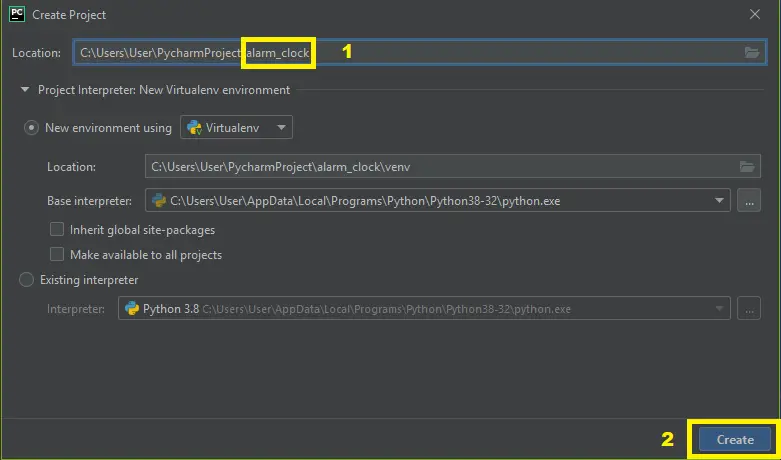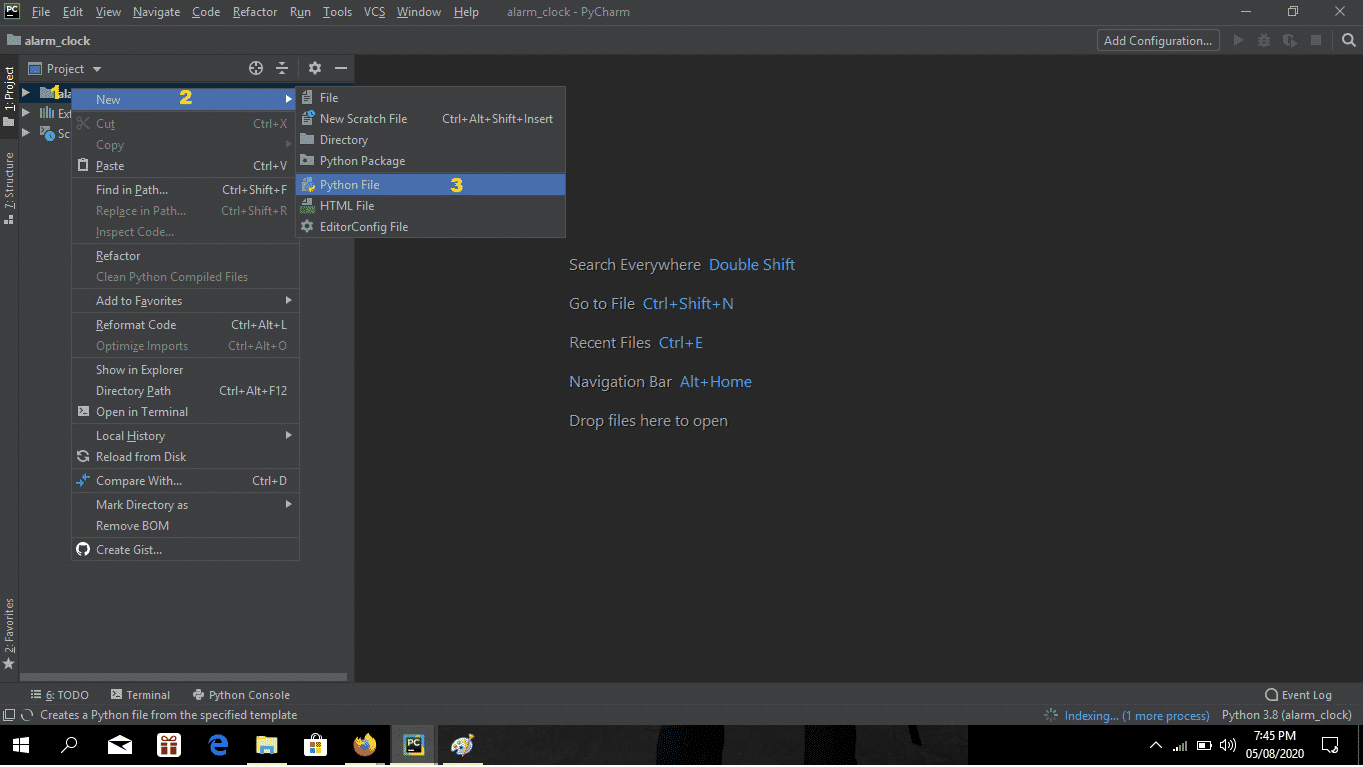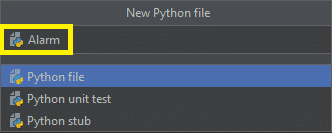# Alarm Clock Using Python With Source Code

The Alarm Clock Using Python consists of very useful libraries such as datetime and tkinter which help us to build project using the current date and time. Apart from this, they provide a user interface to set the alarm according to the requirement in 24-hour format.

## Alarm Clock Using Python : Project Information

This Python Alarm Clock also includes a downloadable Python Alarm Clock Code for free. To start creating this simple project Alarm Clock Using Python, make sure that you have Pycharm IDE installed in your computer.

By the way if you are new to python programming and you don’t know what would be the the Python IDE to use, I have here a list of Best Python IDE for Windows, Linux, Mac OS that will suit for you. I also have here How to Download and Install Latest Version of Python on Windows.

## Steps on How To Make An Alarm Clock in Python.

Time needed: 5 minutes.

These are the steps on how to create an Alarm Clock Using Python With Source Code

• Step 1: Create a project name.

First open Pycharm IDE and then create a “project name” after creating a project name click the “create” button.• Step 2: Create a python file.

Second after creating a project name, “right click” your project name and then click “new” after that click the “python file“.• Step 3: Name your python file.

Third after creating a python file, Name your python file after that click “enter“.• Step 4: The actual code.

You are free to copy the code given below and download the full source code below.

## Code Explanation

### 1. The Code Given Below Is For Importing Modules.

Code:

``````from tkinter import *
import datetime
import time
import winsound``````

Explanation:

In the given code which is importing all module that being called in executing the program.

### 2. The Code Given Below Is For The Module Actual Time.

Code:

``````def actual_time():
set_alarm_timer = f"{hour.get()}:{min.get()}:{sec.get()}"
alarm(set_alarm_timer)``````

Explanation:

In this module which is the actual or the current time that being called when setting the alarm.

### 3. The Code Given Below Is For The Module Of Setting The Alarm.

Code:

``````def alarm(set_alarm_timer):
while True:
time.sleep(1)
current_time = datetime.datetime.now()
now = current_time.strftime("%H:%M:%S")
date = current_time.strftime("%d/%m/%Y")
print("The Set Date is:",date)
print(now)
if now == set_alarm_timer:
print("Time to Wake up")
winsound.PlaySound("sound.mp3",winsound.SND_ASYNC)
break``````

Explanation:

In this module which is the setting of alarm that being executed.

### 4. The Code Given Below Is For The GUI

Code:

``````clock = Tk()
clock.title("DataFlair Alarm Clock")
clock.geometry("400x200")
time_format=Label(clock, text= "Enter time in 24 hour format!", fg="red",bg="black",font="Arial").place(x=60,y=120)
addTime = Label(clock,text = "Hour  Min   Sec",font=60).place(x = 110)
setYourAlarm = Label(clock,text = "When to wake you up",fg="blue",relief = "solid",font=("Helevetica",7,"bold")).place(x=0, y=29)

# The Variables we require to set the alarm(initialization):
hour = StringVar()
min = StringVar()
sec = StringVar()

#Time required to set the alarm clock:
hourTime= Entry(clock,textvariable = hour,bg = "pink",width = 15).place(x=110,y=30)
minTime= Entry(clock,textvariable = min,bg = "pink",width = 15).place(x=150,y=30)
secTime = Entry(clock,textvariable = sec,bg = "pink",width = 15).place(x=200,y=30)

#To take the time input by user:
submit = Button(clock,text = "Set Alarm",fg="red",width = 10,command = actual_time).place(x =110,y=70)

clock.mainloop()
#Execution of the window.``````

Explanation:

In this module which is the design or the graphical user interface or (GUI) of this project.

## Complete Source Code

``````
#Importing all the necessary libraries to form the alarm clock:
from tkinter import *
import datetime
import time
import winsound

def alarm(set_alarm_timer):
while True:
time.sleep(1)
current_time = datetime.datetime.now()
now = current_time.strftime("%H:%M:%S")
date = current_time.strftime("%d/%m/%Y")
print("The Set Date is:",date)
print(now)
if now == set_alarm_timer:
print("Time to Wake up")
winsound.PlaySound("sound.mp3",winsound.SND_ASYNC)
break

def actual_time():
set_alarm_timer = f"{hour.get()}:{min.get()}:{sec.get()}"
alarm(set_alarm_timer)

clock = Tk()
clock.title("DataFlair Alarm Clock")
clock.geometry("400x200")
time_format=Label(clock, text= "Enter time in 24 hour format!", fg="red",bg="black",font="Arial").place(x=60,y=120)
addTime = Label(clock,text = "Hour  Min   Sec",font=60).place(x = 110)
setYourAlarm = Label(clock,text = "When to wake you up",fg="blue",relief = "solid",font=("Helevetica",7,"bold")).place(x=0, y=29)

# The Variables we require to set the alarm(initialization):
hour = StringVar()
min = StringVar()
sec = StringVar()

#Time required to set the alarm clock:
hourTime= Entry(clock,textvariable = hour,bg = "pink",width = 15).place(x=110,y=30)
minTime= Entry(clock,textvariable = min,bg = "pink",width = 15).place(x=150,y=30)
secTime = Entry(clock,textvariable = sec,bg = "pink",width = 15).place(x=200,y=30)

#To take the time input by user:
submit = Button(clock,text = "Set Alarm",fg="red",width = 10,command = actual_time).place(x =110,y=70)

clock.mainloop()
#Execution of the window.

``````